Next: Results Up: Treatment of experimental deficiencies Previous: Monte Carlo simulation: MCB

## Analytical calculation: ANB

The following approximations were used to derive an analytical function from the complicated intensity expression (eq. 4): (i) All two dimensional transversal distributions are assumed to be Gaussian and approximated by azimuthal symmetrical Gaussian. (ii) A mean MS variance is used, averaged over the crystal. (iii) A combined total electron divergence (ED) distribution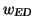from a folding of the MS and BD distributions is used instead. (iv) The variation of gt, being in second order of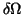and therefore much smaller than the variation in gl, is neglected in the intensity. With that the uncollimated coherent intensity (eq. 4) in terms of the variation lof gl due to ED is expressed as follows: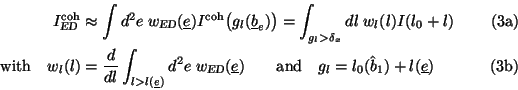The sole dependence of the intensity on l allows the conversion of the two dimensional integral to an integral over l in consideration of the kinematical constraint of the pancake. Eq. 5a has to be calculated numerically but for small BD (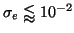) the integral may be solved analytically and will be presented in a forthcoming paper. A collimation function is derived in the following, which includes experimental deficiencies. In the electron coordinate system the circular collimator is characterised by a lateral displacement distribution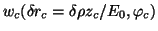of its centre. Due to the azimuthal asymmetry, only the integral over the displacement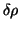has to be calculated. The collimator angle is now a function of the displacement and azimuthal photon angle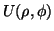, which results in a convolution of the intensity: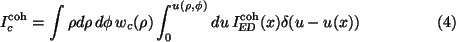Owing to the delta function the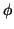integration is trivial and the expression separates into the coherent intensity in terms of the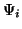functions and a collimation function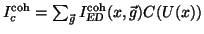. The treatment of collimation in the incoherent case works analogous, but the different angular dependence leads to a remaining integral (note: v=1/(1+Uc2):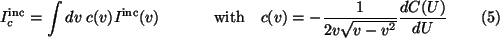Therefore, a single collimation function accounts for experimental deficiencies in both cases of coherent and incoherent bremsstrahlung production. After these derivations, C(U) and c(v) have to be calculated numerically only once and the remaining evaluation of the intensities is a closed analytical calculation providing very fast results.Next: Results Up: Treatment of experimental deficiencies Previous: Monte Carlo simulation: MCB
Frank Natter
1999-07-16